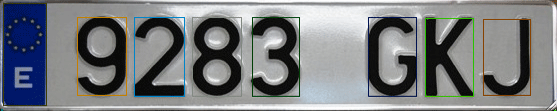In BasiOCR tutorial i explain  how to preprocess, extract features and clasify a handwritten number, and a lot of people ask me how to segment an image where contains several numbers or objects.

In this tutorial I want explain how to segment an image and detect each object inside image, in this tutorial we can detect objects from plate (the numbers) or each object draw into a paper.

We can define three steps to do this task: preprocess image, find countours, and calculate bounding rect. With this three steps we have each object separatly and then we can use each object to classify it with basicocr sample.

For preprocessing task we use:

1. Smooth filter to simple noise elimination.
2. Threshold image to get binary image
3. Morphologic filter, erode and dilate to eliminate noise.Once we have preprocessed our input image we look for the contours in our binary image with cvFindContours, and optimize its with approxpoly.

Then for each searched contour we calculate the bounding rect.

We can now draw his contour and/or bounding rect.Now we have each object differenciate, now we can use this to get characteristics to classificate, for example  the 7 invariant hu moments.

Download source from GitHub